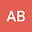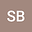Application of alternative Legendre polynomials for solving a class of two-dimensional nonlinear time-fractional diffusion equations of distributed-order
••• AFSHIN BABAEI,
• Sahar Banihashemi
AFSHIN BABAEI
University of Mazandaran
Author ProfileSahar Banihashemi
University of Mazandaran
Author Profile## Abstract

This paper is devoted to finding the numerical solution for a class of nonlinear two-dimensional distributed-order time-fractional diffusion equation. A finite difference scheme is used to discretize the problem in the time direction. Then, a collocation approach based on the alternative Legendre polynomials is employed to solve the discretized nonlinear problem. The numerical algorithm is fully described and convergence analysis of the method is evaluated. Finally, some numerical implementations are provided to highlight the convergence rate and the flexibility of this approach.